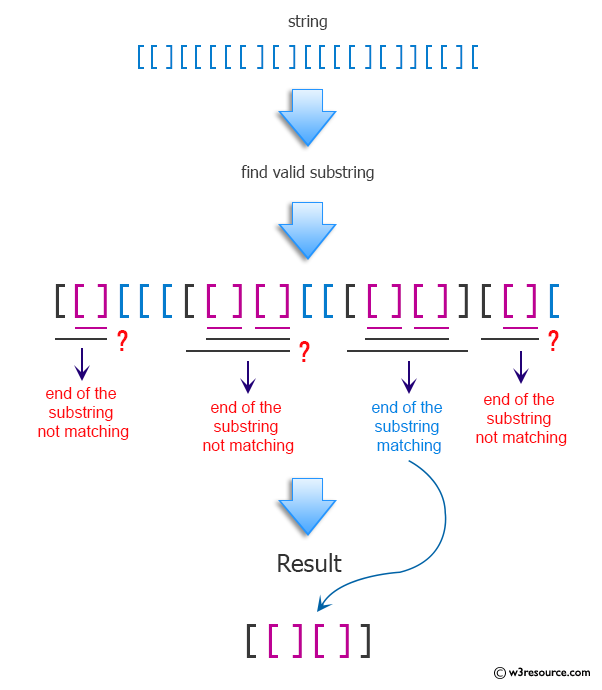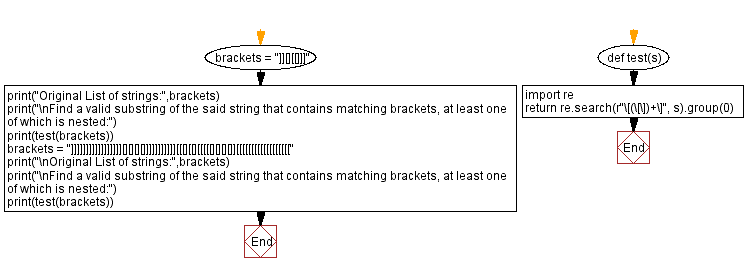﻿ Python: Find a valid substring of s that contains matching brackets, at least one of which is nested - w3resource# Python: Find a valid substring of s that contains matching brackets, at least one of which is nested

## Python Programming Puzzles: Exercise-87 with Solution

Write a Python program to find a valid substring of a given string that contains matching brackets, at least one of which is nested.

```Input:
]][][[]]]

Output:
[[]]

Input:
]]]]]]]]]]]]]]]]][][][][]]]]]]]]]]][[[][[][[[[[][][][]][[[[[[[[[[[[[[[[[[

Output:
[[][][][]]
```

Pictorial Presentation:Sample Solution:

Python Code:

``````#License: https://bit.ly/3oLErEI

def test(s):
import re
return re.search(r"\[(\[\])+\]", s).group(0)

brackets = "]][][[]]]"
print("Original List of strings:",brackets)
print("\nFind a valid substring of the said string that contains matching brackets, at least one of which is nested:")
print(test(brackets))
brackets = "]]]]]]]]]]]]]]]]][][][][]]]]]]]]]]][[[][[][[[[[][][][]][[[[[[[[[[[[[[[[[["
print("\nOriginal List of strings:",brackets)
print("\nFind a valid substring of the said string that contains matching brackets, at least one of which is nested:")
print(test(brackets))
``````

Sample Output:

```Original List of strings: ]][][[]]]

Find a valid substring of the said string that contains matching brackets, at least one of which is nested:
[[]]

Original List of strings: ]]]]]]]]]]]]]]]]][][][][]]]]]]]]]]][[[][[][[[[[][][][]][[[[[[[[[[[[[[[[[[

Find a valid substring of the said string that contains matching brackets, at least one of which is nested:
[[][][][]]
```

Flowchart:## Visualize Python code execution:

The following tool visualize what the computer is doing step-by-step as it executes the said program:

Python Code Editor :

Have another way to solve this solution? Contribute your code (and comments) through Disqus.

What is the difficulty level of this exercise?

Test your Programming skills with w3resource's quiz.

﻿

## Python: Tips of the Day

Clamps num within the inclusive range specified by the boundary values x and y:

Example:

```def tips_clamp_num(num,x,y):
return max(min(num, max(x, y)), min(x, y))
print(tips_clamp_num(2, 4, 6))
print(tips_clamp_num(1, -1, -6))
```

Output:

```4
-1
```